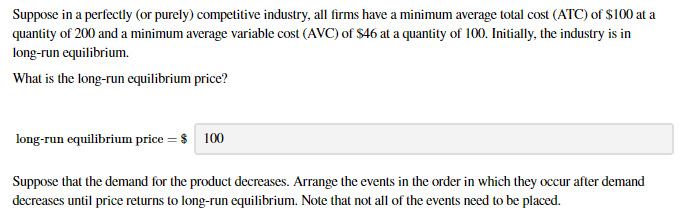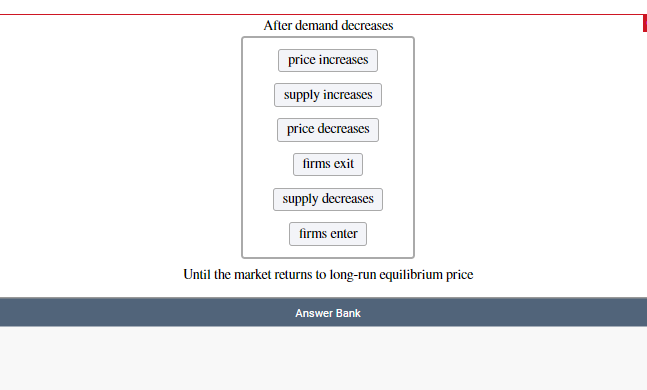# Suppose in a perfectly (or purely) competitive industry, all firms have a minimum average total cost (ATC) of \$100 at aquantity of 200 and a minimum average variable cost (AVC) of \$46 at a quantity of 100. Initially, the industry is inlong-run equilibrium.What is the long-run equilibrium price?long-run equilibrium price = \$100Suppose that the demand for the product decreases. Arrange the events in the order in which they occur after demanddecreases until price returns to long-run equilibrium. Note that not all of the events need to be placed. After demand decreasesprice increasessupply increasesprice decreasesfirms exitsupply decreasesfirms enterUntil the market returns to long-run equilibrium priceAnswer Bank

Question
1 viewshelp_outlineImage TranscriptioncloseSuppose in a perfectly (or purely) competitive industry, all firms have a minimum average total cost (ATC) of \$100 at a quantity of 200 and a minimum average variable cost (AVC) of \$46 at a quantity of 100. Initially, the industry is in long-run equilibrium. What is the long-run equilibrium price? long-run equilibrium price = \$ 100 Suppose that the demand for the product decreases. Arrange the events in the order in which they occur after demand decreases until price returns to long-run equilibrium. Note that not all of the events need to be placed. fullscreenhelp_outlineImage TranscriptioncloseAfter demand decreases price increases supply increases price decreases firms exit supply decreases firms enter Until the market returns to long-run equilibrium price Answer Bank fullscreen
check_circle

Step 1

The perfect competition, in the long-term earns zero economic profit. Thus, in the long run, firms produce the level of output at which the average total cost is minimum.

Step 2

Hence, the long-run equilibrium price is equal to the minimum average cost at quantity of 200. The long run equilibrium price is equal to \$100. This is shown in the figure below. At point A, the average total cost curve is at its minimum point.

Step 3

When the demand for a product decreases, its price falls due to leftward shift in the demand curve. The price falls so much that the firms are unable to cover their minimum average cost. As a result, many firms exit the industry. Due to fall in the numb...

### Want to see the full answer?

See Solution

#### Want to see this answer and more?

Solutions are written by subject experts who are available 24/7. Questions are typically answered within 1 hour.*

See Solution
*Response times may vary by subject and question.
Tagged in